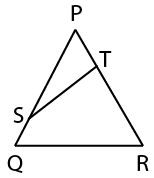Question 93

# In the given figure. QRTS is a cyclic quadrilateral. If PT = 5 cm. SQ= 4 cm. PS = 6 cm and ∠PQR = 63°. then what is the value (in cm) of TR?Solution

∠PQR + ∠STR = 180°

$$\Rightarrow$$ 63° + ∠STR = 180°

$$\Rightarrow$$ ∠STR = 127°

$$\Rightarrow$$ ∠STR + ∠STP = 180°

$$\Rightarrow$$ ∠STP = 180° - 127° = 63°

Triangle PST ~ triangle PQR,

PQ/PT = PR/PS

$$\Rightarrow$$ (PS + SQ)/PT = PR/PS

$$\Rightarrow$$ 10/5 = PR/6

PR = 12

TR = PR - PT = 12 - 5 = 7

• Free SSC Study Material - 18000 Questions
• 230+ SSC previous papers with solutions PDF